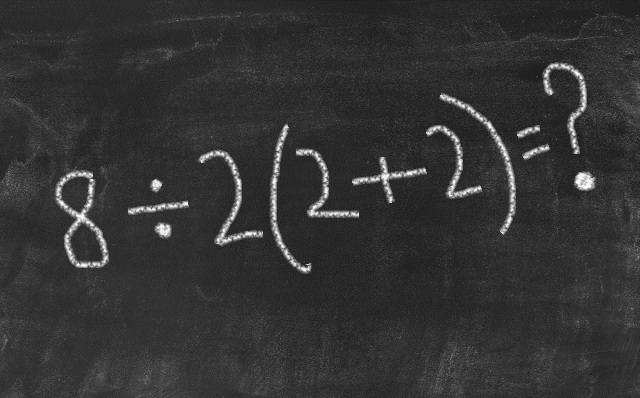# Viral Math equation 8 ÷ 2(2 + 2) divides the internet, can you solve it?

Viral Math equation: Viral math equation 8÷2 (2+2) =? has divided the internet with two possible answers. Check out the correct answer along with the solution.

Created On: Aug 2, 2019 14:33 ISTModified On: Aug 2, 2019 14:37 ISTTwitter math problem simple Math sum divides the internet

Viral Math equation:  Viral math equation 8 ÷ 2(2 + 2) divided the internet, after a twitter user posted it on Monday, July 29. The math equation, which though looks simple, had two conflicting results. While some claim that the answer is 1, others are confident that the answer to the viral math equation is 16. Check out the equation and see for yourself which one is the correct answer.

Twitter math problem:Twitter math problem:

Like Playing with Numbers: This is one of the famous books by Shakuntala Devi!

Math equation: 8÷2 (2+2) =?

First Answer= 1

Second Answer=16

The difference between the two answers is due to two different math rules taught at school- BODMAS and PEMDAS.

BODMAS: Brackets, Orders, Division, Multiplication, Addition, Subtraction

PEMDAS: Parentheses, Exponents, Multiplication, Division, Addition, Subtraction

If one follows BODMAS rule, the final answer you get is 1, if you follow PEMDAS rule the final answer you get is 16.

Puzzles to Puzzle You: Recommended Book for You

Twitter math problem: Let us see the explanation of both the answers, BODMAS answer explanation:

PEMDAS answer explanation:

Twitter math problem: Debate

While both sides are convinced that their answer is the correct one, a twitter user has explained that the solution to the math problem depends on mathematician you listen to, because the order of operations isn't a hard and fast rule, and math is just a language.

So what do you think is the correct answer?

Take Weekly Tests on app for exam prep and compete with others. Download Current Affairs and GK app

एग्जाम की तैयारी के लिए ऐप पर वीकली टेस्ट लें और दूसरों के साथ प्रतिस्पर्धा करें। डाउनलोड करें करेंट अफेयर्स ऐप

AndroidIOS
Comment ()

2 + 6 =
Post